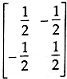# A (-1,1), B (5,3) are opposite vertices of a square in the x - y plane. The equation of the other diagonal ( not passing through A, B ) of the square is given by

1.  x - 3y + 4 = 0

2.  2x - y + 3 = 0

3.  y + 3x - 8 = 0

4.  x + 2y - 1 = 0

4

y + 3x - 8 = 0

Explanation :
No Explanation available for this question

# The sub-tangent, ordinate and sub-normal to the parabola y2 = 4ax at a point ( diffferent from the origin ) are in

1.  A.P.

2.  H.P.

3.  G.P.

4.  None

4

G.P.

Explanation :
No Explanation available for this question

# The set matrices

1.

2.

3.

4.

4Explanation :
No Explanation available for this question

# Volume of the parallelopiped whose coterminal edges are

1.  5 units

2.  6 units

3.  7 units

4.  8 units

4

7 units

Explanation :
No Explanation available for this question

1.  x a x-1 - x b x-1

2.  log (a/b)

3.  log (b/a)

4.  does not exist

4

log (a/b)

Explanation :
No Explanation available for this question

# For the equation

1.  there is only one root

2.  there are only two distinct roots

3.  there are only three distinct roots

4.  there are four distinct roots

4

there are only two distinct roots

Explanation :
No Explanation available for this question

# If ω is a complex cube root of unity then ( 1 - ω + ω2)6 + ( 1- ω2 + ω)6 =

1.  0

2.  6

3.  64

4.  128

4

128

Explanation :
No Explanation available for this question

# The term in the independent of x in the expansion of (3+2x)44, is

1.  4th term

2.  5th term

3.  1st term

4.  7th term

4

1st term

Explanation :
No Explanation available for this question

# If (1+i)100= 249(x+iy), then x2+y2 is equal to

1.  0

2.  4

3.  8

4.  16

4

4

Explanation :
No Explanation available for this question

1.  π/2

2.  π/3

3.  π/4

4.  π/6

4